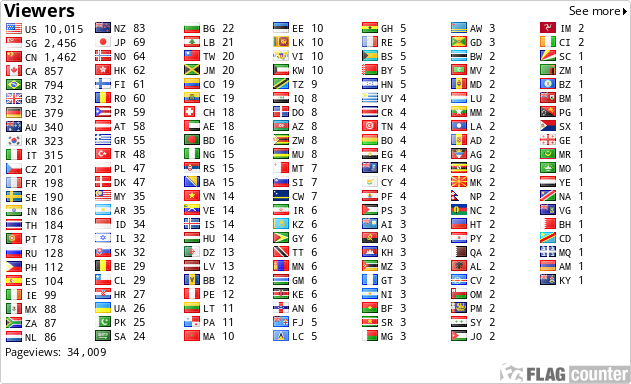# Ohm's Law Power Calculator

Wind and Sun Power: Ohm's Law Power Calculator

WindAndSunPower.com
Ohm's Law Power Calculator
Enter any two values and press Calculate:

 Volts (Volts) Resistance (Ohms) Current (Amps) Power (Watts)

Example of how to use.

1. Enter Volts = Maximum voltage your load will see. Example: Equalization voltage of 15.5V.

2. Enter Resistance = Ohm value of the load your considering using. Example: 2 Ohm's.

3. Press Calculate

4. Results Current = The current or amps your load will draw at the voltage and Ohm value entered.

5. Results Power = The Minimum Watts your load needs to be rated for at the voltage and Ohm value entered.